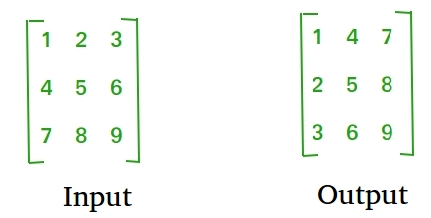Skip to content
Related Articles
Java Program to find transpose of a matrix
• Last Updated : 07 Nov, 2018

Transpose of a matrix is obtained by changing rows to columns and columns to rows. In other words, transpose of A[][] is obtained by changing A[i][j] to A[j][i].For Square Matrix :

The below program finds transpose of A[][] and stores the result in B[][], we can change N for different dimension.

 `// Java Program to find ``// transpose of a matrix`` ` `class` `GFG``{``    ``static` `final` `int` `N = ``4``;``     ` `    ``// This function stores transpose``    ``// of A[][] in B[][]``    ``static` `void` `transpose(``int` `A[][], ``int` `B[][])``    ``{``        ``int` `i, j;``        ``for` `(i = ``0``; i < N; i++)``            ``for` `(j = ``0``; j < N; j++)``                ``B[i][j] = A[j][i];``    ``}``     ` `    ``// Driver code``    ``public` `static` `void` `main (String[] args)``    ``{``        ``int` `A[][] = { {``1``, ``1``, ``1``, ``1``},``                      ``{``2``, ``2``, ``2``, ``2``},``                      ``{``3``, ``3``, ``3``, ``3``},``                      ``{``4``, ``4``, ``4``, ``4``}};``     ` `        ``int` `B[][] = ``new` `int``[N][N], i, j;``     ` `        ``transpose(A, B);``     ` `        ``System.out.print(``"Result matrix is \n"``);``        ``for` `(i = ``0``; i < N; i++)``        ``{``            ``for` `(j = ``0``; j < N; j++)``            ``System.out.print(B[i][j] + ``" "``);``            ``System.out.print(``"\n"``);``        ``}``    ``}``}`` ` `// This code is contributed by Anant Agarwal.`
Output:

```Result matrix is
1 2 3 4
1 2 3 4
1 2 3 4
1 2 3 4
```

For Rectangular Matrix :

The below program finds transpose of A[][] and stores the result in B[][].

 `// Java Program to find ``// transpose of a matrix`` ` `class` `GFG``{``    ``static` `final` `int` `M = ``3``;``    ``static` `final` `int` `N = ``4``;``     ` `    ``// This function stores transpose``    ``// of A[][] in B[][]``    ``static` `void` `transpose(``int` `A[][], ``int` `B[][])``    ``{``        ``int` `i, j;``        ``for` `(i = ``0``; i < N; i++)``            ``for` `(j = ``0``; j < M; j++)``                ``B[i][j] = A[j][i];``    ``}``     ` `    ``// Driver code``    ``public` `static` `void` `main (String[] args)``    ``{``        ``int` `A[][] = { {``1``, ``1``, ``1``, ``1``},``                      ``{``2``, ``2``, ``2``, ``2``},``                      ``{``3``, ``3``, ``3``, ``3``}};``     ` `        ``int` `B[][] = ``new` `int``[N][M], i, j;``     ` `        ``transpose(A, B);``     ` `        ``System.out.print(``"Result matrix is \n"``);``        ``for` `(i = ``0``; i < N; i++)``        ``{``            ``for` `(j = ``0``; j < M; j++)``            ``System.out.print(B[i][j] + ``" "``);``            ``System.out.print(``"\n"``);``        ``}``    ``}``}`` ` `// This code is contributed by Anant Agarwal.`
Output:
```Result matrix is
1 2 3
1 2 3
1 2 3
1 2 3
```

In-Place for Square Matrix:

 `// Java Program to find ``// transpose of a matrix`` ` `class` `GFG``{``    ``static` `final` `int` `N = ``4``;``     ` `    ``// Finds transpose of A[][] in-place``    ``static` `void` `transpose(``int` `A[][])``    ``{``        ``for` `(``int` `i = ``0``; i < N; i++)``            ``for` `(``int` `j = i+``1``; j < N; j++)``            ``{``                 ``int` `temp = A[i][j];``                 ``A[i][j] = A[j][i];``                 ``A[j][i] = temp;``            ``}``    ``}``     ` `    ``// Driver code``    ``public` `static` `void` `main (String[] args)``    ``{``        ``int` `A[][] = { {``1``, ``1``, ``1``, ``1``},``                      ``{``2``, ``2``, ``2``, ``2``},``                      ``{``3``, ``3``, ``3``, ``3``},``                      ``{``4``, ``4``, ``4``, ``4``}};``        ` `        ``transpose(A);``     ` `        ``System.out.print(``"Modified matrix is \n"``);``        ``for` `(``int` `i = ``0``; i < N; i++)``        ``{``            ``for` `(``int` `j = ``0``; j < N; j++)``            ``System.out.print(A[i][j] + ``" "``);``            ``System.out.print(``"\n"``);``        ``}``    ``}``}`
Output:
```Modified matrix is
1 2 3 4
1 2 3 4
1 2 3 4
1 2 3 4
```

Please refer complete article on Program to find transpose of a matrix for more details!

My Personal Notes arrow_drop_up